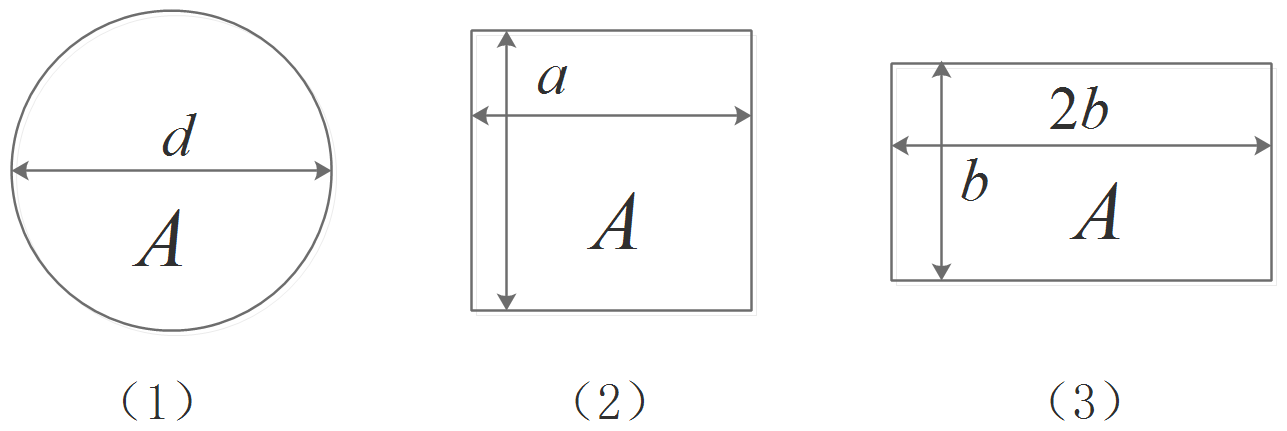# 第6章 流动阻力和能量损失--课后作业【含答案】 水力学

（1）单选题

A  对

B  错

（2）单选题

A  对

B  错

（3）单选题

A为边长为a的正方形截面管道，B为半径为a的圆管，已知两管长度相同，通过流量相同，沿程水头损失系数相同，则两管的沿程水头损失（ ）

A  hfA大于hfB

B  hfA等于hfB

C  hfA小于hfB

D  尚不能确定大小

（4）单选题

A  对

B  错

（5）单选题

A  对

B  错

（6）单选题

A  增大

B  减小

C  不变

D  不定

（7）单选题

A、B两根圆形输水管，管径相同，雷诺数相同，A管为热水，B管为冷水，则两管流量（ ）

A  QA大于QB

B  QA等于QB

C  QA小于QB

D  不能确定

（8）单选题A  $\tau _{01} 大于 \tau _{02} 大于 \tau _{03}, Q_1大于Q_2大于Q_3$

B  $\tau _{01} 小于 \tau _{02} 小于 \tau _{03}, Q_1小于Q_2小于Q_3$

C  $\tau _{01} 大于 \tau _{02} 大于 \tau _{03}, Q_1小于Q_2小于Q_3$

D  $\tau _{01} 小于 \tau _{02} 小于 \tau _{03}, Q_1大于Q_2大于Q_3$

（9）单选题

A  $\tau _{01} : \tau _{02} : \tau _{03} = 1.0:0.75:0.5, Q_1:Q_2:Q_3=1.0:0.75:0.5$

B  $\tau _{01} : \tau _{02} : \tau _{03} = 1.0:0.886:0.837, Q_1:Q_2:Q_3=1.0:0.886:0.837$

C  $\tau _{01} : \tau _{02} : \tau _{03} = 1.0:0.886:0.837, Q_1:Q_2:Q_3=1.0:0.9416:0.915$

D  $\tau _{01} : \tau _{02} : \tau _{03} = 1.0:0.9416:0.915, Q_1:Q_2:Q_3=1.0:0.9416:0.915$

（10）单选题

A  断面流速分布规律相同

B  断面上切应力分布规律相同

C  断面上压强分布规律相同

D  沿程阻力与水头损失规律相同

（11）单选题

A  $v\sqrt{\frac{\lambda}{8}}$

B  $\frac{\rho v^2}{2}$

C  $v\sqrt{\frac{8}{\lambda}}$

D  $\lambda \frac{\rho v^2}{8}$

（12）单选题

A  0.0666m

B  0.169m

C  0.357m

D  无法确定

（13）单选题

A  0.3m/s

B  0.45m/s

C  0.6m/s

D  0.9m/s

（14）单选题

A  对

B  错

（15）单选题

A  对

B  错

（16）多选题

A  边界阻力

B  因流层相对运动而产生的时均粘性切应力

C  时均流速变化引起的紊流附加切应力

D  质点掺混引起的动量交换导致的紊流附加切应力

（17）单选题

A  管壁绝对粗糙度

B  管壁相对粗糙度

C  粘性底层厚度与管壁绝对粗糙度之比

D  雷诺数

（18）单选题

A  对

B  错

（19）单选题

A  两者相等

B  前者大于后者

C  前者小于后者

D  无法确定